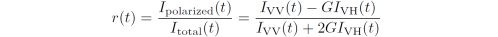### The G-factor in time-resolved fluorescence anisotropy

In time-resolved fluorescence anisotropy, the sample is excited using vertically polarized light pulses while the intensity decay of the sample is measured through a polarizer oriented vertically, VV, and horizontally, VH, to the sample. The anisotropy decay, r(t), is then calculated aswere G is the instrument sensitivity ratio towards vertically and horizontally
polarized light. In other words, a G-factor of e.g. G = 2 means that the detection system detects vertically polarized light "twice as good" as horizontally polarized light.

G is measured by exciting the sample using horizontally polarized light and subsequently measuring the horizontally and vertically polarized components of the emission intensity (HH and HV), each for the same period of time. Since there is no difference between the number of photons coming towards the HH and HV channels from the sample, G is calculated as the ratio between the measured total intensities (counts) in each channel:Note that some software use the opposite definition of the G-factor (i.e. G = HH/HV). In AniFit the G-factor must be supplied manually.

### Setting the G-factor in AniFit

The G-factor is set in the editbox next to the IRFs listbox.

When setting the G-factor, the entered value is applied to all loaded data that currently has a G-factor of G = 1 (the default). In order to set individual G-factors for each data-set simply select the data set from the Decays listbox and enter the corresponding G-factor in the G-factor Editbox. Note here how the G-factor value in the editbox is automatically updated when selecting a new data set from the Decays listbox.